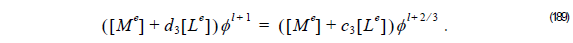Using recurrence formula Equation 118 for Padé (3,3), we get: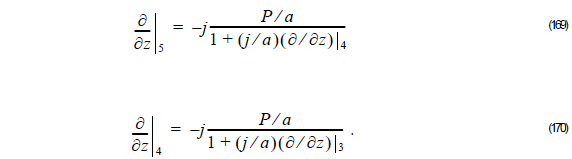Using Equation 145 into Equation 169, we get:Finally, using Equation 171 into Equation 169, we get:Here a = 2k0nre .

From Equation 172, we get:Thus,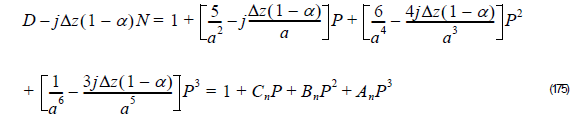and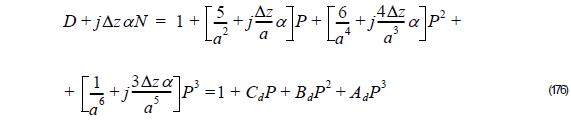where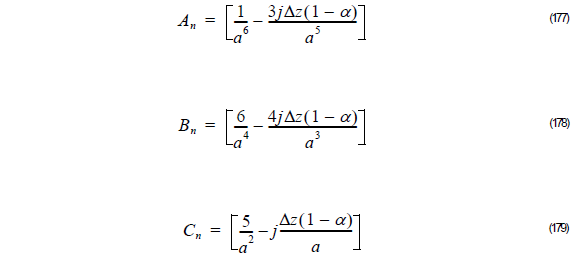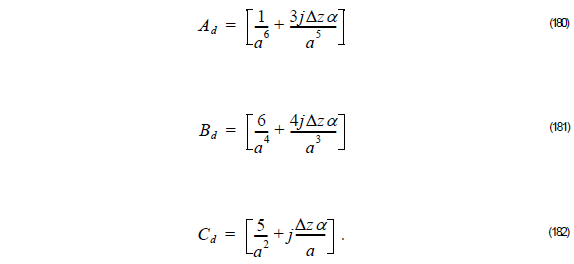Thus, the unknown field φ l + 1  at z + Δz is related to the known field φ l at z as follows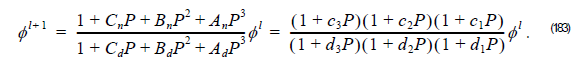In order to solve Equation 183, we use the Multistep method, that is, the unknown field

φ l + 1  can be obtained from the known field φ l  by successively solving Equation 164 for

= 1, 2, 3 . Therefore for Padé(3,3) we follow the steps:

1          Using φ l , compute φ l + 1 ⁄ 3  considering the linear system: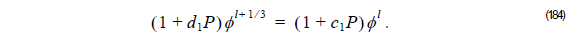In case of using FEM solve,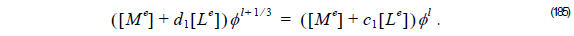2          Using φ l+ 1 ⁄ 3, compute φ l + 2 ⁄ 3 considering the linear system: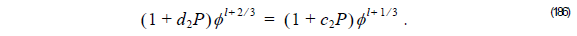In case of using FEM compute,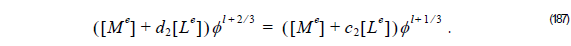3          Finally, using φ l+ 2 ⁄ 3, compute the following linear system to obtain the unknown field φ l + 1  at z + Δz :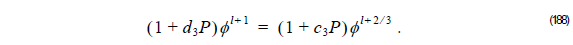In case of using FEM compute,